## How to Calculate and Solve for Well Bore Storage Factor, Fluid Density and Well Annulus Cross-Sectional Area in Well Testing | The Calculator Encyclopedia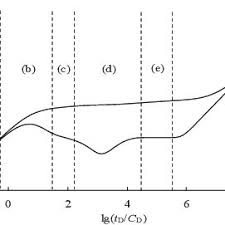The image above represents the well bore storage factor.

To compute for the well bore storage factor, two essential parameters are needed and these parameters are Well Annulus Cross-Sectional Area (Ao) and Fluid Density (ρ).

The formula for calculating the well bore storage factor:

C = 144Ao / 5.615ρ

Where;

C = Well Bore Storage Factor
Ao = Well Annulus Cross-Sectional Area
ρ = Fluid Density

Let’s solve an example;
Find the well bore storage factor when the well annulus cross-sectional area is 54 and fluid density is 42.

This implies that;

Ao = Well Annulus Cross-Sectional Area = 54
ρ = Fluid Density = 42

C = 144Ao / 5.615ρ
C = 144 x 54 / 5.615 x 42
C = 7776/235.83
C = 32.97

Therefore, the well bore storage factor is 32.97.

Calculating the Well Annulus Cross-Sectional Area when the Well bore Storage Factor and the Fluid Density is Given.

Ao = C x 5.615ρ / 144

Where;

Ao = Well Annulus Cross-Sectional Area
C = Well Bore Storage Factor
ρ = Fluid Density

Let’s solve an example;
Find the well annulus cross-sectional area when the well bore storage factor is 34 and fluid density is 24.

This implies that;

C = Well Bore Storage Factor = 34
ρ = Fluid Density = 24

Ao = C x 5.615ρ / 144
Ao = 34 x 5.615 x 24 / 144
Ao = 4581.84 / 144
Ao = 31.81

Therefore, the well annulus cross-sectional area is 31.81.

## How to Calculate and Solve for Crude Oil Gravity, Oil Density and Water Density | The Calculator Encyclopedia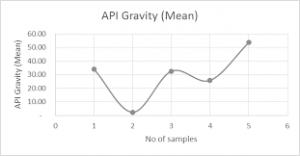The image above represents crude oil gravity.

To compute for the crude oil gravity, two essential parameters are needed and these parameters are oil density (ρo) and water density (ρw).

The formula for calculating the crude oil gravity:

γo = ρo / ρw

Where;

γo = Crude Oil Gravity
ρo = Oil Density
ρw = Water Density

Let’s solve an example;
Find the crude oil gravity when the oil density is 28 and the water density is 46.

This implies that;

ρo = Oil Density = 28
ρw = Water Density = 46

γo = ρo / ρw
γo = 28 / 46
γo = 0.608

Therefore, the crude oil density is 0.608.

Calculating the Oil Density when the Crude Oil Gravity and the Water Density is Given.

ρo = γo x ρw

Where;

ρo = Oil Density
γo = Crude Oil Gravity
ρw = Water Density

Let’s solve an example;
Find the oil density when the crude oil gravity is 30 and the water density is 16.

This implies that;

γo = Crude Oil Gravity = 30
ρw = Water Density = 16

ρo = γo x ρw
ρo = 30 x 16
ρo = 480

Therefore, the oil density is 480.

## How to Calculate and Solve for Total Production Time, Stabilized Well Flow, Cumulative Production before Shut-In | Well Testing | Nickzom Calculator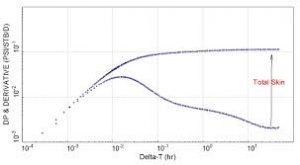The image represents the total production time.

To compute for the total production time, two essential parameters are needed and these parameters are Cumulative Production before Shut-In (Np) and Stabilized Well Flow (Qo).

The formula for calculating the total production time:

tp = 24Np / Qo

Where:

tp = Total Production Time
Np = Cumulative Production before Shut-In
Qo = Stabilized Well Flow

Let’s solve an example;
Find the total production time when the cumulative production before Shut-In is 24 and the stabilized well flow is 43.

This implies that;

Np = Cumulative Production before Shut-In = 24
Qo = Stabilized Well Flow = 43

tp = 24Np / Qo
tp = 24 x 24 / 43
tp = 576 / 43
tp = 13.39

Therefore, the total production time is 13.39 hrs.

## How to Calculate and Solve for the Radius of Investigation in Well Testing | The Calculator Encyclopedia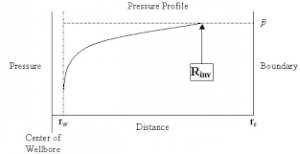The image above represents radius of investigation.

To compute for the radius of investigation, five essential parameters are needed and these parameters are permeability (k), porosity (φ), viscosity (μ), time (t) and total compressibility (CT).

The formula for calculating the radius of investigation:

rinv = 0.0325 √[Kt / φμCT]

Where:

K = Permeability
φ = Porosity
μ = Viscosity
t = Time
CT = Total Compressibility

Let’s solve an example;
Find the radius of investigation when the permeability is 25, porosity is 18, viscosity is 12, time is 22 and total compressibility is 37.

This implies that;

K = Permeability = 25
φ = Porosity =18
μ = Viscosity = 12
t = Time = 22
CT = Total Compressibility = 37

rinv = 0.0325 √[Kt / φμCT]
rinv = 0.0325 √[25 x 22 / 18 x 12 x 37]
rinv = 0.0325 √[25 x 22 / 7992]
rinv = 0.0325 √[550 / 7992]
rinv = 0.0325 √[0.068]
rinv = 0.0325 [0.26]
rinv = 0.0085

Therefore, the radius of investigation is 0.0085 ft.

## How to Calculate and Solve for Infinite Acting Period | The Calculator Encyclopedia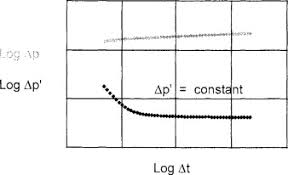The image represents the infinite acting period.

To compute the infinite acting period, six essential parameters are needed and these parameters are permeability (k), porosity (φ), well drainage area (A), viscosity (μ), Dimensionless Time to End of Infinite Acting Period ((tDA)eia) and total compressibility (CT).

The formula for calculating the infinite acting period:

teia = [φ μ CT A / 0.000263K] (tDA)eia

Where;

teia = Infinite Acting Period
K = Permeability
φ = Porosity
A = Well Drainage Area
μ = Viscosity
(tDA)eia = Dimensionless Time to End of Infinite Acting Period
CT = Total Compressibility

Let’s solve an example;
Given that the permeability is 21, porosity is 15, well drainage area is 32, viscosity is 26, dimensionless time to end of infinite acting period is 44 and total compressibility is 34.
Find the infinite acting period?

This implies that;

K = Permeability = 21
φ = Porosity = 15
A = Well Drainage Area = 32
μ = Viscosity = 26
(tDA)eia = Dimensionless Time to End of Infinite Acting Period = 44
CT = Total Compressibility = 34

teia = [φ μ CT A / 0.000263K] (tDA)eia
teia = [15 x 26 x 34 x 32 / 0.000263 x 21] 44
teia = [424320 / 0.005523] 44
teia = [76827810.972] 44
teia = 3380423682.78

Therefore, the infinite acting period is 3380423682.78 s.

## How to Calculate and Solve for Dimensionless Time in Well Testing | The Calculator Encyclopedia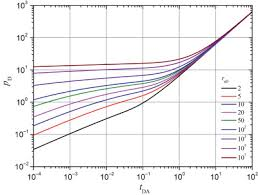The image above represents dimensionless time.

To compute for the dimensionless time, six essential parameters are needed and these parameters are permeability (k), porosity (φ), viscosity (μ), well radius (rw), minimum transient time (t) and total compressibility (CT).

The formula for calculating dimensionless time:

tD = 0.000263kt / φμCTr

Where;

tD = Dimensionless Time
k = Permeability
φ = Porosity
μ = Viscosity
t = Minimum Transient Time
CT = Total Compressibility

Let’s solve an example
Find the dimensionless time when the permeability is 13, porosity is 11.5, viscosity is 10.5, well radius is 24, minimum transient time is 17 with a total compressibility of 28.

This implies that;

k = Permeability = 13
φ = Porosity = 11.5
μ = Viscosity = 10.5
rw = Well Radius = 24
t = Minimum Transient Time = 17
CT = Total Compressibility = 28

tD = 0.000263kt / φμCTr
tD = 0.000263 x 13 x 1711.5 x 10.5 x 28 x 24²
tD = 0.000263 x 13 x 1711.5 x 10.5 x 28 x 576
tD = 0.000263 x 13 x 171947456
tD = 0.0581231947456
tD = 2.9845e-8

Therefore, the dimensionless time is 2.98

Nickzom Calculator – The Calculator Encyclopedia is capable of calculating the dimensionless time.

To get the answer and workings of the dimensionless time using the Nickzom Calculator – The Calculator Encyclopedia. First, you need to obtain the app.

You can get this app via any of these means:

To get access to the professional version via web, you need to register and subscribe for NGN 1,500 per annum to have utter access to all functionalities.
You can also try the demo version via https://www.nickzom.org/calculator

Apple (Paid) – https://itunes.apple.com/us/app/nickzom-calculator/id1331162702?mt=8
Once, you have obtained the calculator encyclopedia app, proceed to the Calculator Map, then click on Petroleum under EngineeringNow, Click on Well Testing under Petroleum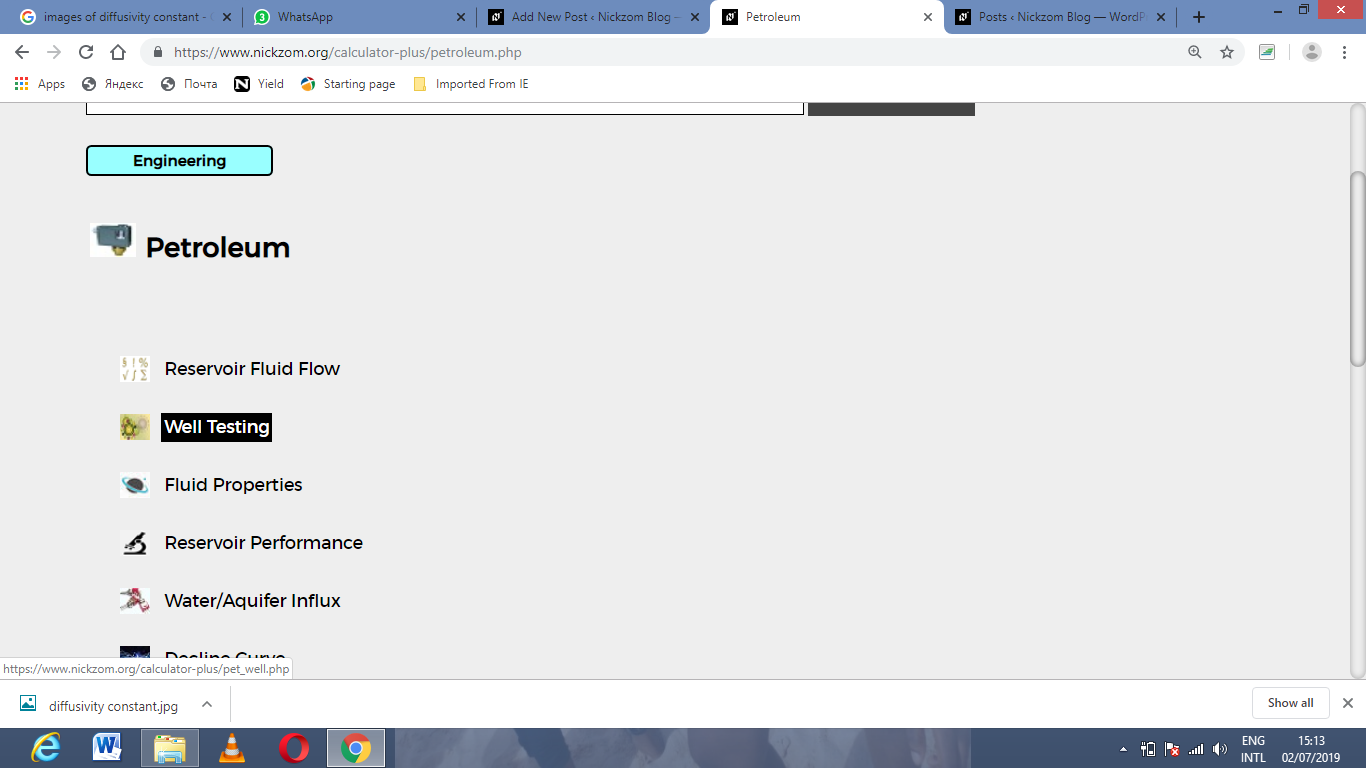Now, Click on Dimensionless Time under Well TestingThe screenshot below displays the page or activity to enter your value, to get the answer for the dimensionless time according to the respective parameter which are the permeability (k), porosity (φ), viscosity (μ), well radius (rw), minimum transient time (t) and total compressibility (CT).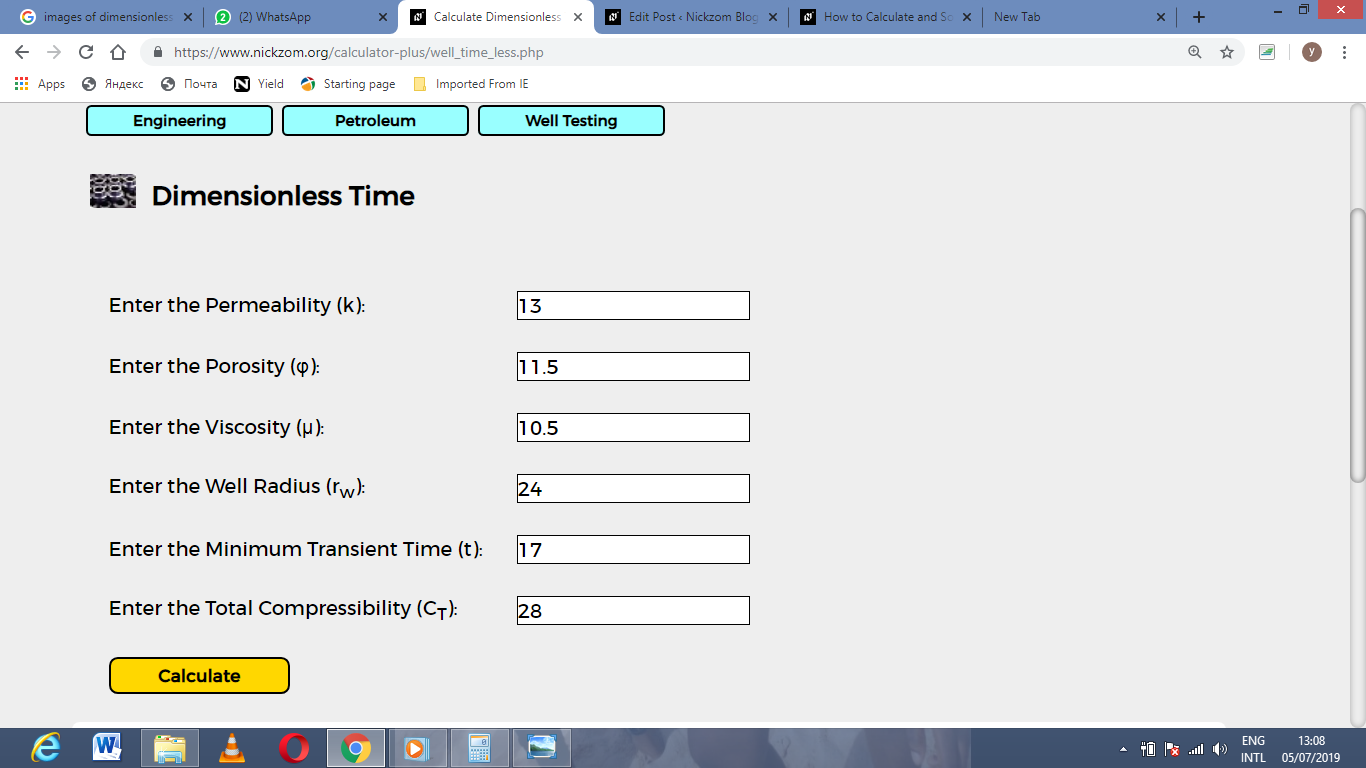Now, enter the value appropriately and accordingly for the parameter as required by the permeability (k) is 13, porosity (φ) is 11.5, viscosity (μ) is 10.5, well radius (rw) is 24, minimum transient time (t) is 17 and total compressibility (CT) is 28.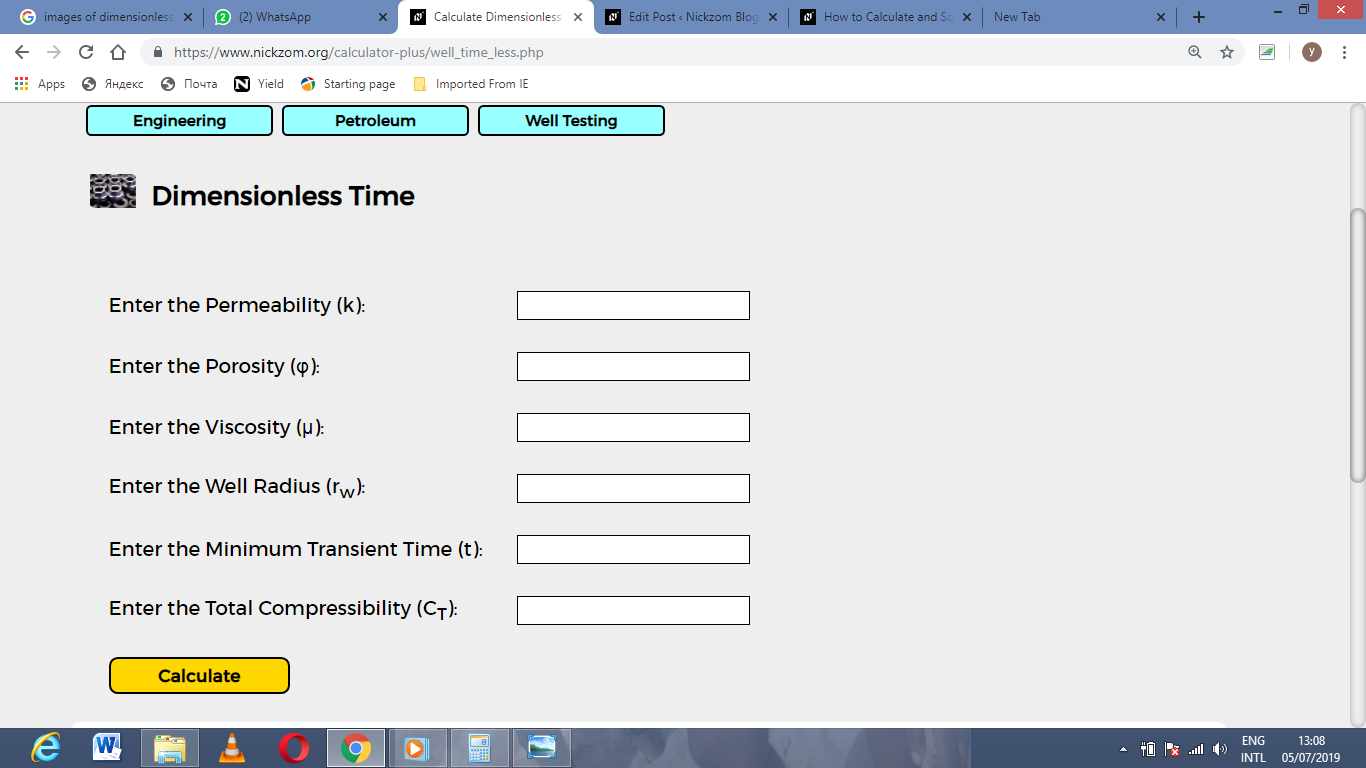Finally, Click on Calculate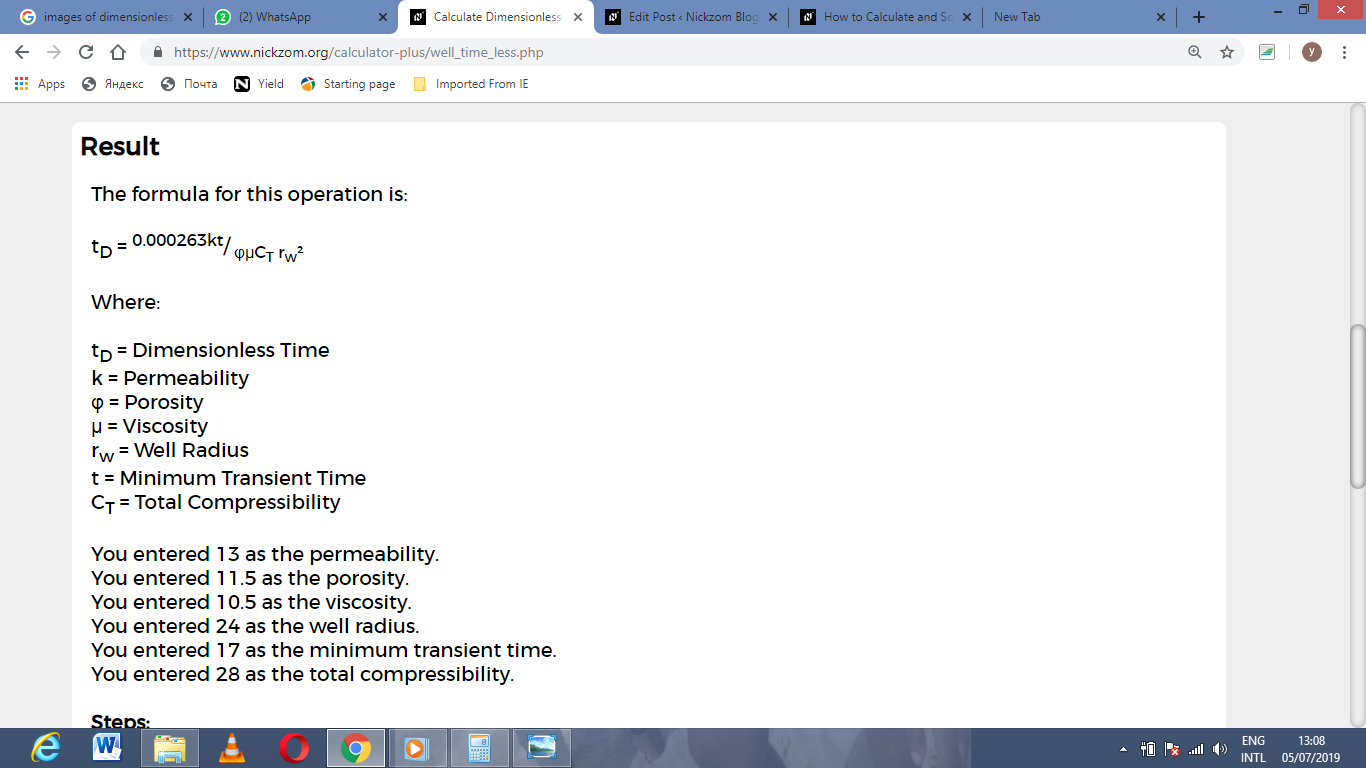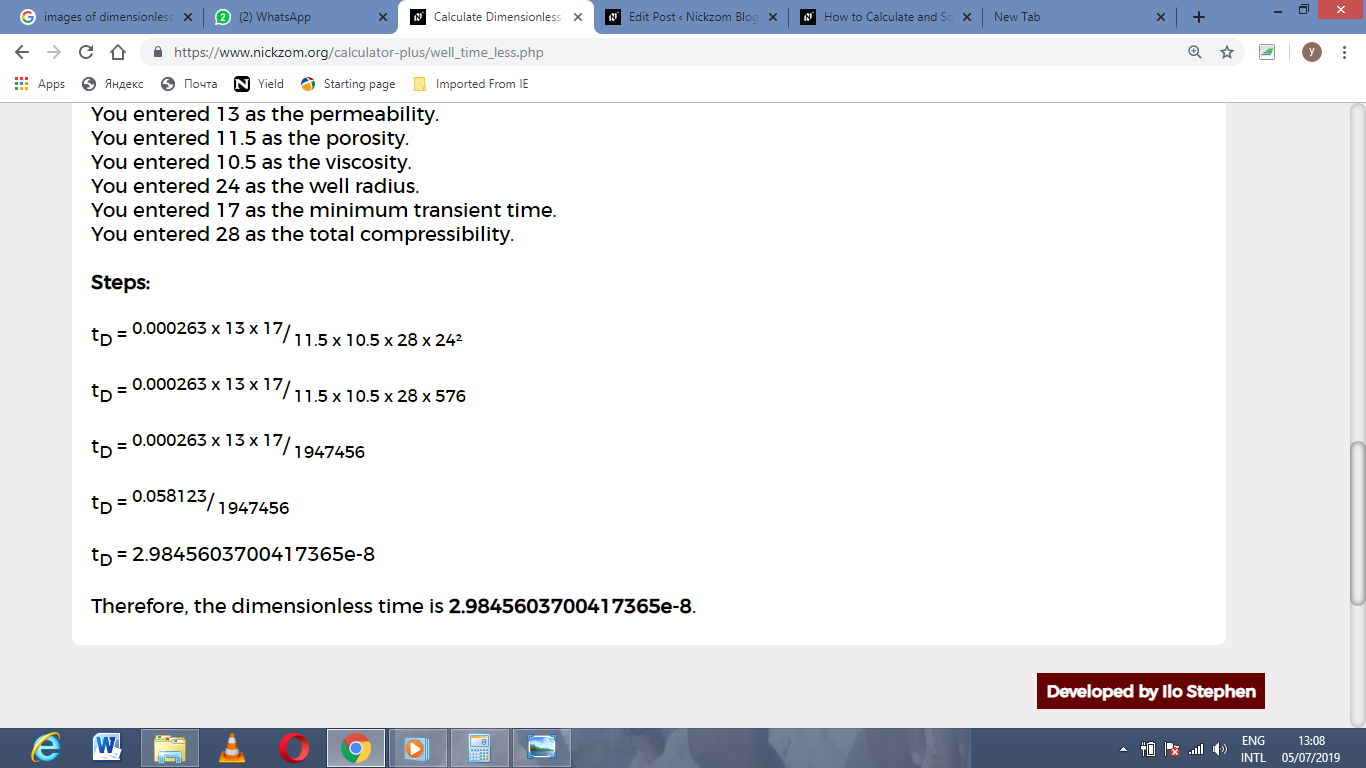As you can see from the screenshot above, Nickzom Calculator– The Calculator Encyclopedia solves for the dimensionless time and presents the formula, workings and steps too.

## How to Calculate and Solve for Minimum Transient Time in Well Testing | The Calculator Encyclopedia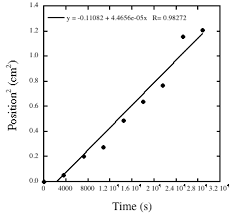The image above represents minimum transient time.

To compute for the minimum transient time, five essential parameters are needed and these parameters are permeability (k), porosity (φ), reservoir radius (r), viscosity (μ) and total compressibility (CT).

The formula for calculating the minimum transient time:

t = 9.48 x 104 φ CT r² / k

Where;

t = Minimum Transient Time
k = Permeability
φ = Porosity
μ = Viscosity
CT = Total Compressibility

Let’s solve an example;
Given that the permeability is 11, porosity is 14, reservoir radius is 7, viscosity is 13 and total compressibility is 22.
Find the minimum transient time?

This implies that;

k = Permeability = 11
φ = Porosity = 14
r = Reservoir Radius = 7
μ = Viscosity = 13
CT = Total Compressibility = 22

t = 9.48 x 104 φ CT r² / k
t = 9.48 x 104 x 14 x 22 x 7² / 11
t = 9.48 x 104 x 14 x 22 x 49 / 11
t = 1430721600 / 11
t = 130065600

Therefore, the minimum transient time is 13006500.

## How to Calculate and Solve for Diffusivity Constant | Well Testing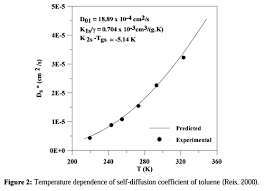The image above represents diffusivity constant.

To compute for the diffusivity constant, four essential parameters are needed and these parameters are pemerability (k), porosity (φ), viscosity (μ) and total compressibility (CT).

The formula for calculating diffusivity constant:

η = 0.000263k / φμCT

Where;

η = Diffusivity Constant
k = Permeability
φ = Porosity
μ = Viscosity
CT = Total Compressibility

Let’s solve an example;
Find the diffusivity constant where the permeability is 12, porosity is 9, viscosity is 7 and total compressibility is 11.

This implies that;

k = Permeability = 12
φ = Porosity = 9
μ = Viscosity = 7
CT = Total Compressibility = 11

η = 0.000263k /  φμCT
η = 0.000263 x 12 / 9 x 7 x 11
η = 0.003156 / 693
η = 0.000004554112554112554

Therefore, the diffusivity constant is 0.000004554112554112554.

Calculating the Permeability when the Diffusivity Constant, Porosity, Viscosity and Total Compressibility is Given.

k = n x φμCT / 0.000263

Where;

k = Permeability
η = Diffusivity Constant
φ = Porosity
μ = Viscosity
CT = Total Compressibility

Let’s solve an example;
Find the permeability where the diffusivity constant is 24, porosity is 10, viscosity is 5 and total compressibility is 13.

This implies that;

η = Diffusivity Constant = 24
φ = Porosity = 10
μ = Viscosity = 5
CT = Total Compressibility = 13

k = n x φμCT / 0.000263
k = 24 x 10 x 5 x 13 / 0.000263
k = 15600 / 0.000263
k = 59315589.3536

Therefore, the permeability is 59315589.3536.

Calculating the Porosity when the Diffusivity Constant, Permeability, Viscosity and Total Compressibility is Given.

φ = 0.000263k / nμCT

Where;

φ = Porosity
k = Permeability
η = Diffusivity Constant
μ = Viscosity
CT = Total Compressibility

Let’s solve an example;
Find the porosity where the diffusivity constant is 28, permeability is 8, viscosity is 12 and total compressibility is 18.

This implies that;

k = Permeability = 8
η = Diffusivity Constant = 28
μ = Viscosity = 12
CT = Total Compressibility = 18

φ = 0.000263k / nμCT
φ = 0.000263 x 8 / 28 x 12 x 18
φ = 0.002104 / 6048
φ = 3.478e-7

Therefore, the porosity is 3.47e-7.

## How to Calculate and Solve for Gas Pore Volume of a Reservoir Fluid Flow | The Calculator Encyclopedia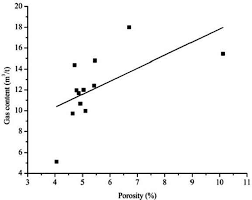The image above represents the gas pore volume.

To compute for the gas pore volume, three essential parameters are needed and these parameters are Initial Gas FVF (Bgi), Initial Water Saturation (Swi) and Initial Gas in Place (G).

The formula for calculating the gas pore volume:

(PV)g = GBgi / 1 – Swi

Where;

(PV)g = Gas Pore Volume
Bgi = Initial Gas FVF
Swi = Initial Water Saturation
G = Initial Gas in Place

Let’s solve an example;
Find the gas pore volume when the initial gas FVF is 12, initial water saturation is 9 and initial gas in place is 13.

This implies that;

Bgi = Initial Gas FVF = 12
Swi = Initial Water Saturation = 9
G = Initial Gas in Place = 13

(PV)g = GBgi / 1 – Swi
(PV)g = 13 x 12 / 1 – 9
(PV)g = 156 / -8
(PV)g =  -19.5

Therefore, the gas pore volume is -19.5

Calculating the Initial Gas FVF when the Gas Pore Volume, Initial Water Saturation and Initial Gas in Place is Given.

Bgi = (PV)g (1 – Swi) / G

Where;

Bgi = Initial Gas FVF
(PV)g = Gas Pore Volume
Swi = Initial Water Saturation
G = Initial Gas in Place

Let’s solve an example;
Find the initial gas FVF when the gas pore volume is 19, initial water saturation is 11 and initial gas in place is 16.

This implies that;

(PV)g = Gas Pore Volume = 19
Swi = Initial Water Saturation = 11
G = Initial Gas in Place = 16

Bgi = (PV)g (1 – Swi) / G
Bgi = 19 (1 – 11) / 16
Bgi = 19 (-10) / 16
Bgi = -190 / 16
Bgi = -11.875

Therefore, the initial gas FVF is -11.875.

Calculating the Initial Water Saturation when the Gas Pore Volume, Initial Gas FVF and Initial Gas in Place is Given.

Swi = 1 – GBgi / (PV)g

Where;

Swi = Initial Water Saturation
G = Initial Gas in Place
(PV)g = Gas Pore Volume
Bgi = Initial Gas FVF

Let’s solve an example;
Find the initial water saturation when the initial gas FVF is 24, initial gas in place is 6 and gas pore volume is 46.

This implies that;

G = Initial Gas in Place = 6
(PV)g = Gas Pore Volume = 46
Bgi = Initial Gas FVF = 24

Swi = 1 – GBgi / (PV)g
Swi = 1 – 6 x 24 / 46
Swi = 1 – 144 / 46
Swi = 1 – 3.13
Swi = -2.13

Therefore, the initial water saturation is -2.13.

## How to Calculate and Solve for Total Formation Volume Factor | The Calculator Encyclopedia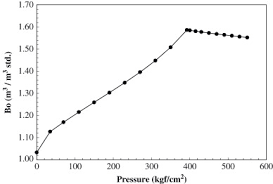The image above represents the total formation volume factor (FVF).

To compute for the total FVF, four essential parameters are needed and these parameters are oil FVF (Bo), gas FVF (Bg), initial gas solubility (Rsi) and current gas solubility (Rs).

The formula for calculating the total FVF:

BT = Bo + (Rsi – Rs)Bg

Where;

BT = Total FVF
Bo = Oil FVF
Bg = Gas FVF
Rsi = Initial Gas Solubility
Rs = Current Gas Solubility

Let’s solve an example;
Find the total FVF when the oil FVF is 14, gas FVF is 15, initial gas solubility is 26 and current gas solubility is 17.

This implies that;

Bo = Oil FVF = 14
Bg = Gas FVF = 15
Rsi = Initial Gas Solubility = 26
Rs = Current Gas Solubility = 17

BT = Bo + (Rsi – Rs)Bg
BT = 14 + (26 – 17)15
BT = 14 + (9)15
BT = 14 + 135
BT = 149

Therefore, the total FVF is 149.

Calculating the Oil FVF when the Total FVF, Gas FVF, Initial Gas Solubility and Current Gas Solubility is Given.

Bo = BT – (Rsi – Rs)Bg

Where;

Bo = Oil FVF
BT = Total FVF
Bg = Gas FVF
Rsi = Initial Gas Solubility
Rs = Current Gas Solubility

Let’s solve an example;
Find the oil FVF when the total FVF is 44, gas FVF is 17, initial gas solubility is 21 and current gas solubility is 19.

This implies that;

BT = Total FVF = 44
Bg = Gas FVF = 17
Rsi = Initial Gas Solubility = 21
Rs = Current Gas Solubility = 19

Bo = BT – (Rsi – Rs)Bg
Bo = 44 – (21 – 19)17
Bo = 44 – (2)17
Bo = 44 – 34
Bo = 10

Therefore, the oil FVF is 10.

Calculating the Gas FVF when the Total FVF, Oil FVF, Initial Gas Solubility and Current Gas Solubility is Given.

Bg = BT – Bo / Rsi – Rs

Where;

Bg = Gas FVF
Bo = Oil FVF
BT = Total FVF
Rsi = Initial Gas Solubility
Rs = Current Gas Solubility

Let’s solve an example;
Find the gas FVF when the total FVF is 48, oil FVF is 16, initial gas solubility is 20 and current gas solubility is 12.

This implies that;

Bo = Oil FVF = 16
BT = Total FVF = 48
Rsi = Initial Gas Solubility = 20
Rs = Current Gas Solubility = 12

Bg = BT – Bo / Rsi – Rs
Bg = 48 – 16 / 20 – 12
Bg = 32 / 8
Bg = 4

Therefore, the gas FVF is 4.